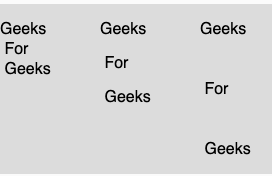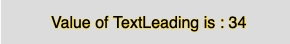• Last Updated : 17 Apr, 2019

The textAlign() function in p5.js is used to set the spacing, in pixels, between lines of text. This function is used in all subsequent calls to the text() function.

Syntax:

`textLeading(leading)`

Parameters: This function accepts single argument leading which stores the size in pixels for spacing between the lines.

Below programs illustrate the textLeading() function in p5.js:

Example 1: This example uses textAlign() function to set the spacing, in pixels, between lines of text.

 `function` `setup() {`` ` `    ``// Create Canvas of given size``    ``createCanvas(380, 170);``}`` ` `function` `draw() {``    ``let string = ``"Geeks \n For \n Geeks"``;``     ` `    ``// Set the background color``    ``background(220);``     ` `    ``// Set the text size``    ``textSize(16);``     ` `    ``// Set the text ``    ``text(string, 0, 30);``     ` `    ``// set the text leading``    ``textLeading(34)``     ` `    ``text(string, 100, 30);``     ` `    ``// set the text leading``    ``textLeading(60)``     ` `    ``text(string, 200, 30);``}`

Output:Example 2: This example uses textAlign() function to return the spacing, in pixels, between lines of text.

 `function` `setup() {`` ` `    ``// Create Canvas of given size``    ``createCanvas(380, 170);``}`` ` `function` `draw() {``     ` `    ``let string = ``"Geeks \n For \n Geeks"``;``     ` `    ``// Set the background color``    ``background(220);``     ` `    ``// Set the text size``    ``textSize(16);``     ` `    ``// Set the text leading``    ``textLeading(34)``     ` `    ``// Get the value of text leading``    ``var` `u = textLeading();``     ` `    ``// Set the stroke color``    ``stroke(255, 204, 0);``     ` `    ``// Display the value``    ``text(``"Value of TextLeading is : "` `+ u, 50, 30);``}`

Output:My Personal Notes arrow_drop_up